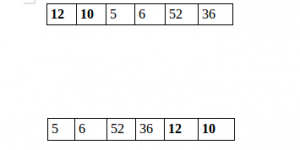# Python Program to Split the array and add the first part to the end

There is a given an array and split it from a specified position, and move the first part of array add to the end.Examples:

```Input : arr[] = {12, 10, 5, 6, 52, 36}
k = 2
Output : arr[] = {5, 6, 52, 36, 12, 10}
Explanation : Split from index 2 and first
part {12, 10} add to the end .

Input : arr[] = {3, 1, 2}
k = 1
Output : arr[] = {1, 2, 3}
Explanation : Split from index 1 and first
```

## Recommended: Please try your approach on {IDE} first, before moving on to the solution.

 `# Python program to split array and move first ` `# part to end. ` ` `  `def` `splitArr(arr, n, k):  ` `    ``for` `i ``in` `range``(``0``, k):  ` `        ``x ``=` `arr[``0``] ` `        ``for` `j ``in` `range``(``0``, n``-``1``): ` `            ``arr[j] ``=` `arr[j ``+` `1``] ` `         `  `        ``arr[n``-``1``] ``=` `x ` `         `  ` `  `# main ` `arr ``=` `[``12``, ``10``, ``5``, ``6``, ``52``, ``36``] ` `n ``=` `len``(arr) ` `position ``=` `2` ` `  `splitArr(arr, n, position) ` ` `  `for` `i ``in` `range``(``0``, n):  ` `    ``print``(arr[i], end ``=` `' '``) ` ` `  `# Code Contributed by Mohit Gupta_OMG <(0_o)>    `

Output:

```5 6 52 36 12 10
```

Please refer complete article on Split the array and add the first part to the end for more details!

Another Solution :

 `# Python program to split array and move first ` `# part to end. ` ` `  `def` `splitArr(a, n, k):  ` `   ``b ``=` `a[:k] ` `   ``return` `(a[k::]``+``b[::]) ` `         `  ` `  `# main ` `arr ``=` `[``12``, ``10``, ``5``, ``6``, ``52``, ``36``] ` `n ``=` `len``(arr) ` `position ``=` `2` `arr ``=` `splitArr(arr, n, position) ` `for` `i ``in` `range``(``0``, n):  ` `    ``print``(arr[i], end ``=` `' '``) `

Output:

```5 6 52 36 12 10
```

My Personal Notes arrow_drop_up

Improved By : ishagup14

Article Tags :

1

Please write to us at contribute@geeksforgeeks.org to report any issue with the above content.